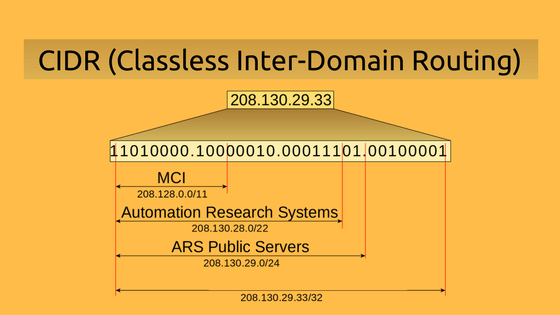Blog Amazon Web Services What is CIDR (Classless Inter-Domain Routing)?# What is CIDR (Classless Inter-Domain Routing)?

## Exam Objective

The topic “CIDR” addresses the Design and Implementation of AWS Networks as highlighted in the AWS Blueprint for the exam guide

[divider /][divider /]

## What is CIDR?

CIDR stands for Classless Inter-Domain Routing and is used for IP addressing and routing. It allocates IP addresses in a more flexible manner as compared to the original system of Internet Protocol (IP) address classes. In this way, it increases the number of available IP addresses with extensive use of NAT (Network Address Translation).

By providing a new, more efficient way to allocate network addresses, CIDR has reduced the issue of wasted address space in the routers. With CIDR, one entry in the routing table entry represents a combination of networks existing in the forward path. This network aggregation in a single address is known as Supernet.

#### CIDR Notation

CIDR IP addresses can be described as consisting of two groups of bits. The most significant group of bits denotes the prefix i.e., a network address that is used for the identification of a network or sub-network. The least significant group of bits is known as host identifier that determines the total number of bits in the address. It is used to signify the device on the work that will receive incoming information packets.

For example, consider the following CIDR Notation

182.0.1.2/28

Here, the prefix is – 182.0.1.2, and

The total number of bits in this address is 28.

#### CIDR Block

The prefix, first group of bits in the notation allows you to group the multiple blocks of network addresses into a single routing network. CIDR blocks share the first group of bits (the binary representation of the network addresses). The blocks are also identified using same decimal dot notation system as IPv4 addresses.

For example, a CIDR block is shown below

10.0.1.0/24

Here /24 signifies the total number of 1’s bits in the routing mask (network mask).

This IP address can be shown as below in the binary format:

11111111.11111111.11111111.00000000

Here the first 24 bits are marked as 1.

It would be equivalent to a network mask of 255.255.255.0

Note that the network addresses that have the identical prefix and the same number of bits, always belong the same block. Also, the large and small blocks can be distinguished by the length of the prefix.

Let’ say that we have given a notation of /20 as the mask, how can we calculate the network mask

We can do this with the help of the below table notation.

 128 64 32 16 8 4 2 1

For a mask of /20, the equivalent binary format would be as follows

11111111.11111111.11110000.11111111

From the third octet, e can see that there are 4 leading 1’s. This corresponds to the first 4 columns of our table

 128 64 32 16 8 4 2 1

If we add these numbers we get – 240

Hence our network mask would be 255.255.240.0

## Calculating the Number of Subnets and Hosts

Now based on a CIDR block, let’s say we need to calculate the number of subnets and hosts possible. We can do this with the following equations.

• Number of Networks possible in a CIDR network, use 2n where n is the number of 1s in the subnet mask
• Number of hosts possible in a CIDR network, use 2n-2 where n is the number of 0s in the host mask
• Every network needs 2 addresses, 1 for network and 1 for broadcast

Let’s consider the following example,

There is a given CIDR block of IP address 192.168.1.0/28

So first let’s get the decimal format in place

11111111.11111111.11111111.11110000

Now, here the first 3 octets are dedicated to the Network

11111111.11111111.11111111.11110000

The first 4 bits of 1’s of the 4th octet is what gives us the possibility of the subnets. So the value of n is 4.

11111111.11111111.11111111.11110000

So the number of subnets is (2*2*2*2) = 16

Next, we need to calculate the number of hosts

Since the number of host bits is 4, hence the value of n is also 4

11111111.11111111.11111111.11110000

So the number of hosts is (2*2*2*2) – 2 = 14

## CIDR for VPC’s and Subnets

• The allowed range of CIDR block size for the VPC (Virtual Private Cloud) is between a /16 network mask (65,536 IP addresses) and /28 network mask (16 IP addresses).
• The CIDR block of a subnet can be same as that of the block for the VPC (for a single subnet in the VPC)
• The CIDR block of a subnet can also be same as that of the other subset in case of multiple subnets.
• In a subnet CIDR block, the first four IP addresses and the last IP address in each subnet block are not available to use, and cannot be assigned to an instance. For example, in a subnet with block 10.0.0.0/24, the following five IP addresses are reserved:
2. 0.0.1: Reserved by AWS for the VPC router.
3. 0.0.2: Reserved by AWS.
4. 0.0.3: Reserved by AWS for future use.